Privacy Statement

2.7 Limits Of Trigonometric Functions

The trigonometric functions have following important limit properties: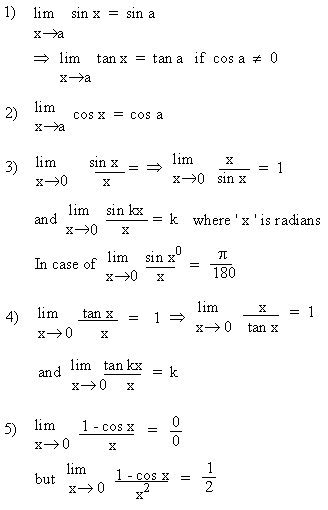Your browser does not support the IFRAME tag.

Example 1
Evaluate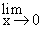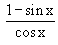Solution :  Let L  =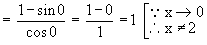Example 2 Evaluate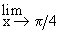( 1 + cot x )

Solution : Let L =( 1 + cot x )

= 1 + cot ( p/4 ) = 1 + 1 = 2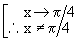Example 3 Evaluate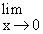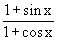Solution : Let L  =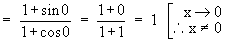Example 4  Evaluate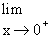cot x

Solution : Let L =cot x    =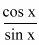Here sin x ® 1 and cos x ® 0 as x ® 0 and x > 0 ( in the 1st quadrant). Therefore the function cot x increases without bound and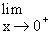cot x = + ¥.

It has a vertical asymptote at x = 0.

Example 5 Evaluate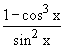Solution : Let

L        =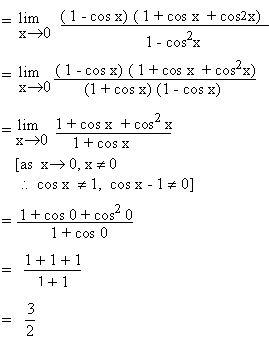Index

2.1 Modulus
2.2 Inequalities
2.3 Limits Of Functions
2.4 Left Hand And Right Hand Limits
2.5 Theorems On The Algebra Of Limits
2.6 Evaluating Limits
2.7 Limits Of Trigonometric Functions
2.8 The Exponential Limits

Chapter 3